# SSC CGL 2013 Tier 1 21 April First Sitting

Instructions

In each of the following questions, which answer figure will complete the question figure?

Question 191

Question Figure :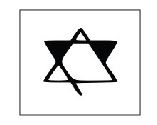Instructions

For the following questions answer them individually

Question 192

Which one of the following box can be created by folding the given key design?
Question Figure :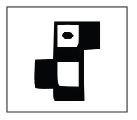Question 193

Which figure represent the relationship among Sun, Moon, Molecule ?

Question 194

Choose from the following diagrams a), b), c) and d) the one that illustrates the relationship among three given classes :
North America, United States of America, New York

Question 195

How many circles are there in the following figure ?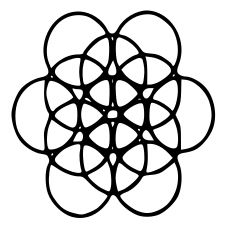Question 196

Three views of the same cube are given. All the faces of the cube are numbered from 1 to 6. Select one figure which will result when the cube is unfolded.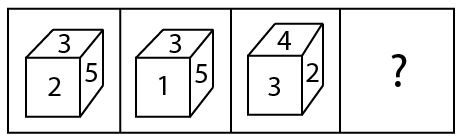Question 197

A word is represented by only one set of numbers as given in any one of the alternatives. The sets of numbers given in the alternatives are represented by two classes of alphabets as in the matrix given below. The columns and rows of Matrix are numbered from 0 to 6. A letter from the matrix can be represented first by its row and next by its column, e,g., ‘A’ can be represented by 42, 62, etc. and ‘P’ can be represented by 15, 43, etc. Similarly, you have to identify the set for the word ‘CALM’.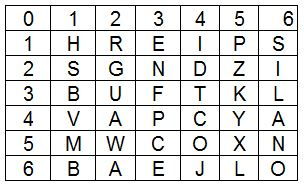Question 198

A piece of paper is folded, and cut as shown below in the question figures. From the given answer figures indicate how it will appear when opened.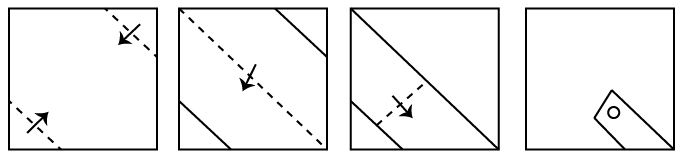Question 199

Which answer figure includes all the components given in the question figure ?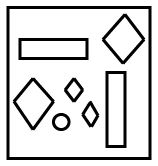Question 200

If a mirror is placed on the line MN, then which of the answer figures is the right
image of the given figure :
Figure :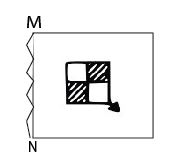OR